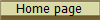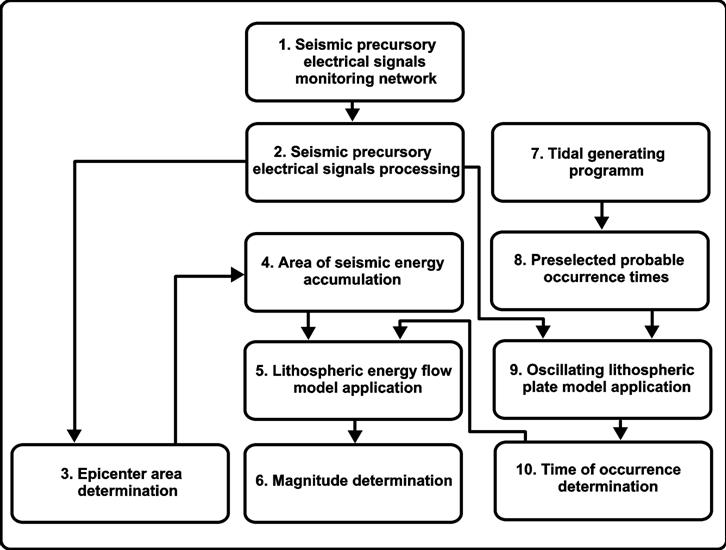Short-term Earthquake Prediction Based on Seismic Precursory Electric  Signals Recorded on Ground Surface.

 CONTENTS 1. 2. 3. 4. 4.1. 4.2. 4.3. 5. 6. 7. 8. 9. 10. 11. 12. 13.6. IMPLEMENTATION OF THE METHOD

The theoretical part of the methodology has already been presented. Moreover, examples of analyzed, real seismic events have been presented, too. An overall flow-chart that shows how all these topics, already dealt with, are integrated in an actually working procedure will be shown in this part of the presentation.
In the following figure (6.1) are given the various steps (numbered accordingly) which are followed in order to have a successful short-term earthquake prediction.Fig. 6.1. Flow-chart, indicating the different, interrelated steps, to be followed, for a successful, short-term earthquake prediction.

The specific flow-chart has two distinct inputs. The first one (step 1) is the monitoring of the Earth's electric field, while the second one (step 7) is the generation of the theoretical, tidal, lithospheric oscillations.
The most probable occurrence times, for a future strong earthquake, are selected, from data which are generated from step (7), at step (8).
From step (1), the seismic precursory electrical signals are processed at step (2) and these signals are correlated at step (9) with the results of step (8). The output of this operation, is the time of occurrence of the future earthquake (step 10), through the use of the oscillating, plate lithospheric model.
From step (2), the epicentral area of the future earthquake is determined at step (3). As an immediate result of this calculation, there is the possibility to calculate the corresponding, cumulative energy which is stored in the regional seismic area as a function of time (step 4) using as input, the past, seismic history of the regional, epicenter area which has already been calculated.
Furthermore, the time of occurrence is already known from step (10) and therefore, the lithospheric, seismic energy flow model can be applied at step (5), using as inputs the output of step (10) and step (4). Consequently, the magnitude (step 6) of the future strong earthquake can be calculated.
In the already followed procedure, just two data inputs were used; namely the seismic, electric, precursory signals and the specific times when max - min oscillating, lithospheric stress-load is achieved.
In conclusion, the three parameters (time, location, magnitude), needed for a successful earthquake prediction, may be determined, following the algorithm, presented, in figure (6.1).